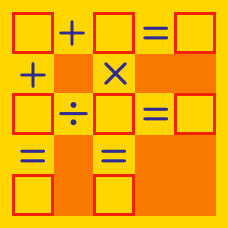Logic

# Arithmetic Puzzles - Operator Search

What series of signs will make the following equation true: $2 \, \square \, 2 \, \square \, 2 \, \square \, 2 = -6$

Which of the following series of signs will make the following equation true: $2 \, \square \, 2 \, \square \, 2 \, \square \, 2 = 4?$

What series of signs will NOT make the following equation true: $8 \, \square \, 8 \, \square \, 8 \, \square \, 8 = 0?$

How many different 3-tuples $(\square,\triangle,\star)$ of operators make the following equation true: $6 \, \square \, (3 \, \triangle \, 2)=1 \, \star \, 1?$

Details and Assumptions

• $\square,\triangle,\star$ can be one of the four arithmetic operators: $+,-,\times,\div.$
• One kind of operator can be used more than once.

What series of signs will NOT make the following equation true: $4 \, \square \, 4 \, \square \, 4 \, \square \, 4 = 16?$

×